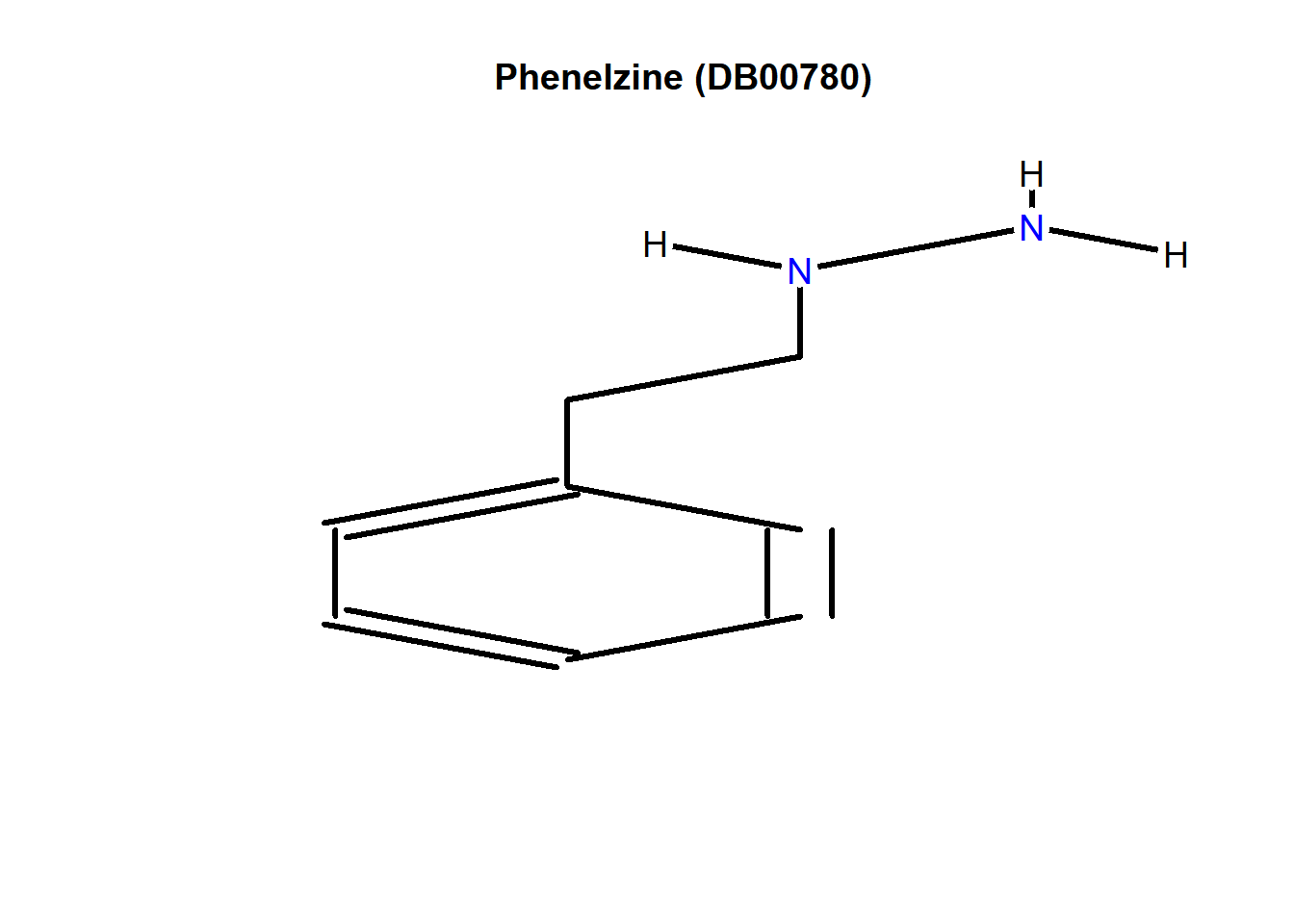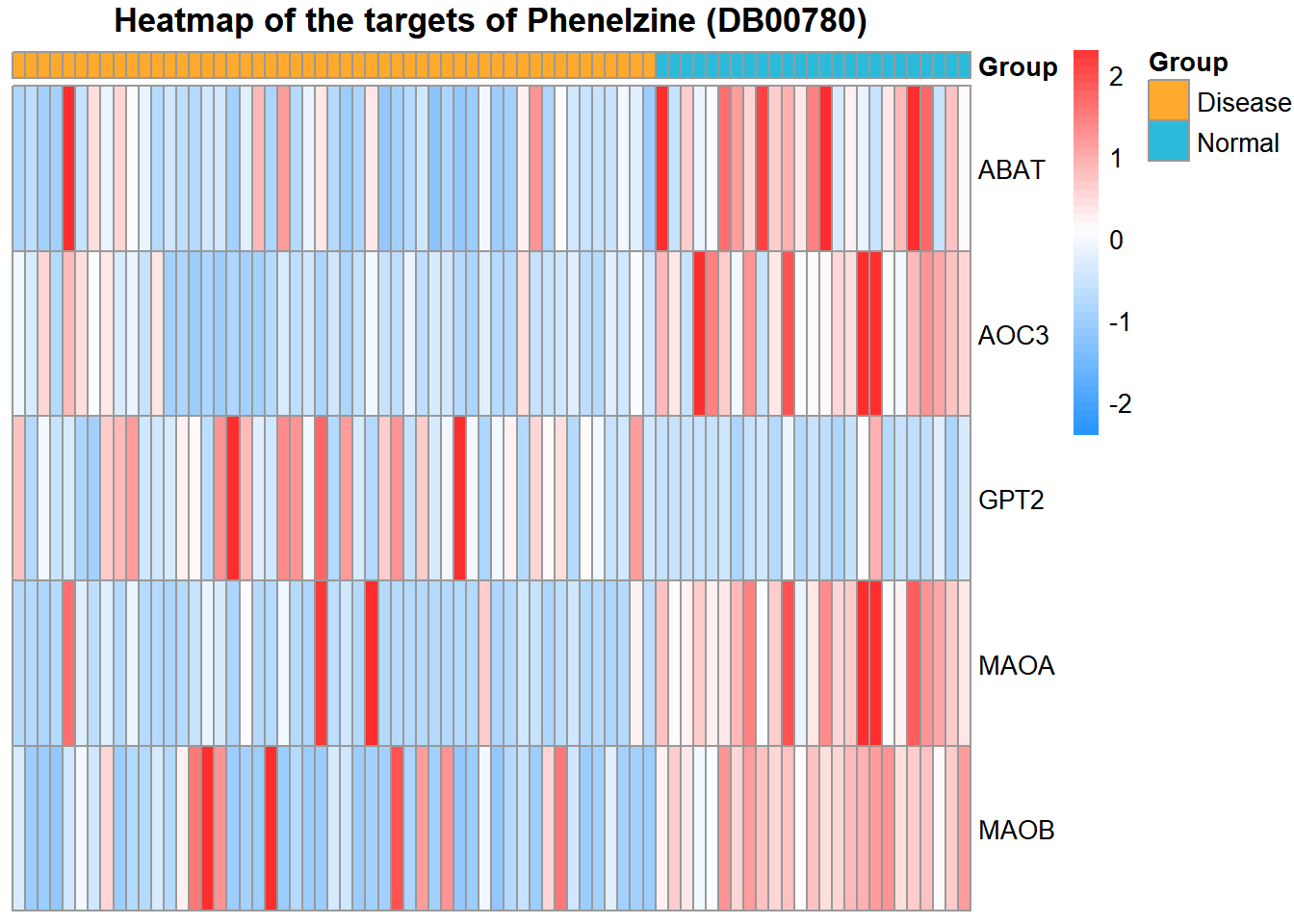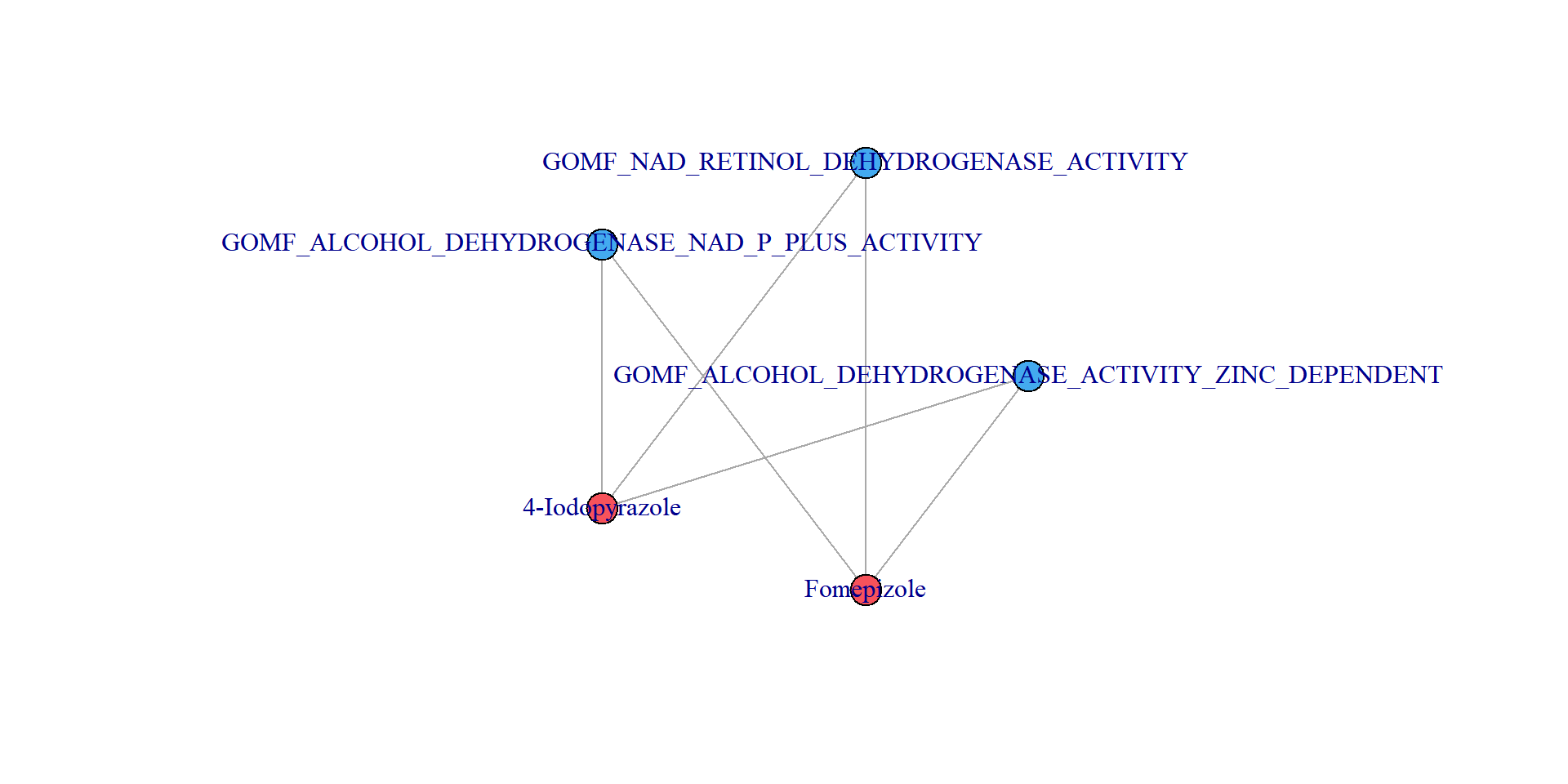## Introduce

This vignette illustrates how to easily use the DrugSim2DR package. Here, we present a network-based computational approach, DrugSim2DR, to perform drug repurposing in specific disease states by integrating drug information, rna-seq data of disease, and GO function data. There are also some functions used to visualize the results

• 1.Calculating the DEscore. We conducted a statistical comparison of gene expression values between case and control groups (e.g. disease and normal, dead and alive). In this case, we use Student’s t-test method to compute the gene differentially expressed level for each gene between disease and normal samples, and convert the t-test p-value of each gene to z-score. The z-score is defined as DEscore, and a larger DEscore indicates the gene regulated by disease to a greater extent.

• 2.Constructing network and performing randomization. In our method, we fist constructed a drug-GO bipartite network. The molecular function gene sets were downloaded from C5 GO gene sets in the Molecular Signatures Database (MSigDB) database (version 7.0). We then defined an edge between a drug and a Go term under the disease status, if they have at least a common gene, and give the weight of this edge that calculate by the Jaccard index and DEscore. Next,we proposed to construct a drug functional similarity network based on the functional similarity between each pair of drugs. The information from the drug-GO bipartite graph was used to evaluate the functional similarity between two drugs as the edge’s weight in the disease context. We consider that functional similarity between two drugs will be stronger when there are more shared GO between the drugs and the more related they are to these GO. Then, we used the eigenvector centrality measure to calculate how central each drug is in this drug-drug functional similarity network. Finally, the statistical significance of these centrality scores is assessed using a bootstrap-based randomization method.

This package provides the Gettest function to return example data set and environment variables, such as the gene expression profile and so on.

### Example 1 : Calculate the DEscore.

The function CalDEscore is used to calculate the DEscore. This function requires users to input the gene expression matrix and the sample label(“1” represents the disease sample. “0” represents the normal sample.). These sample data are stored in this package.

The commands are as follows:

library(DrugSim2DR)
# Obtain the example data
GEP<-Gettest("GEP")
label<-Gettest("label")
# Calculate the zscore
DEscore<-CalDEscore(GEP,label)
#> 3.603273 3.558117 3.794578 8.200240 5.083250

### Example 2 : Calculate drug-drug functional similarity.

The function DrugSimscore is used to calculate drug similarity. According to our method, in this function, the user needs to input DEscore, which is the result of the CalDEscore function. nperm is the number of perturbations The commands are as follows:

# Calculate the centrality score of drugs
drug_similarity<-DrugSimscore(DEscore,nperm = 0)
###view first ten drugs result
drug_similarity[1:5,]


### Example 3 : Identify candidate drugs by random-walk algorithm.

The function DrugReposition is used to repurpose drugs. According to our method, in this function, the user needs to enter the DEscore which is the result of the CalDEscore function. The nperm is the number of perturbations, and the r is the restart probability of random walk. The commands are as follows:

# Calculate the centrality score of drugs
drug_centrality<-DrugReposition(DEscore,nperm = 100,r = 0.9)
###view first ten drugs result
drug_centrality[1:5,]


### Visualize 1: Plot the chemical structure of a compound.

The function plotDrugstructure used to plot the chemical structure of a compound. The user needs to input the drug id. The commands are as follows:

# load depend package
require(ChemmineR)
require(rvest)

# plot the chemical structure
plotDrugstructure("DB00780")### Visualize 2: Plot the heatmap of drug targets expression.

The function plotTargetheatmap can plot the heatmap of drug targets expression. The user needs to input drug id, gene expression profile, and sample label. The commands are as follows:

# load depend package
library('pheatmap')
# plot the heatmap
plotTargetheatmap("DB00780",GEP,label,cluster.rows=FALSE,cluster.cols=FALSE,bk=c(-2.4,2.3),
col=c("#2A95FF","#FF1C1C"))### Visualize 3: Plot a drug/GO bipartite network.

The function plotDruglink can plot a bipartite network of drugs and shared molecular functions. The user needs to input the drugbank ids of drug1 and drug2. The commands are as follows:

# load depend package
library(igraph)
# plot the heatmap
plotDruglink("DB02721","DB01213",i=5)